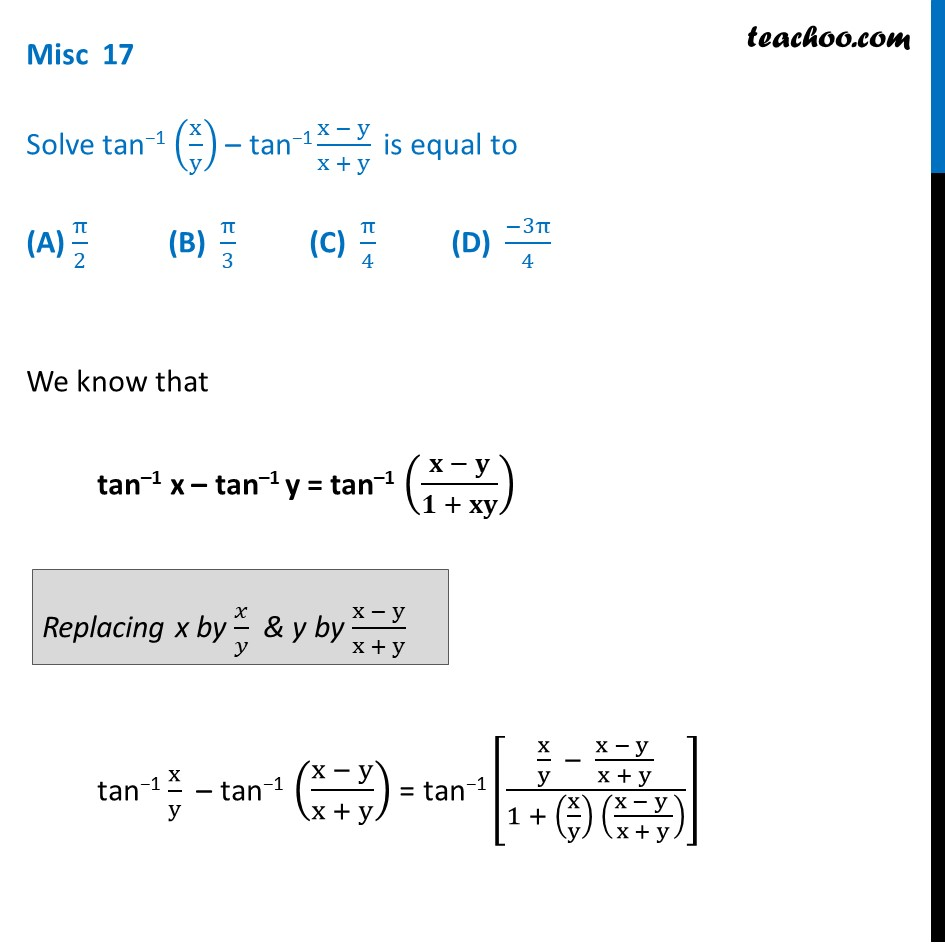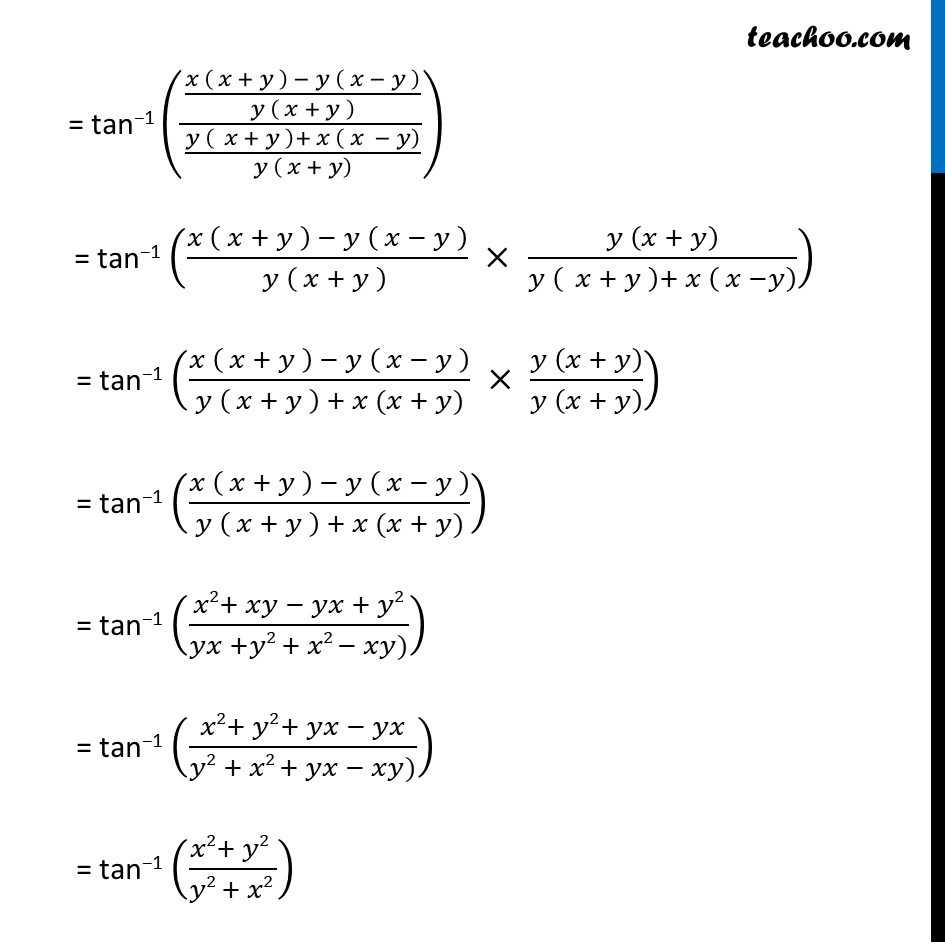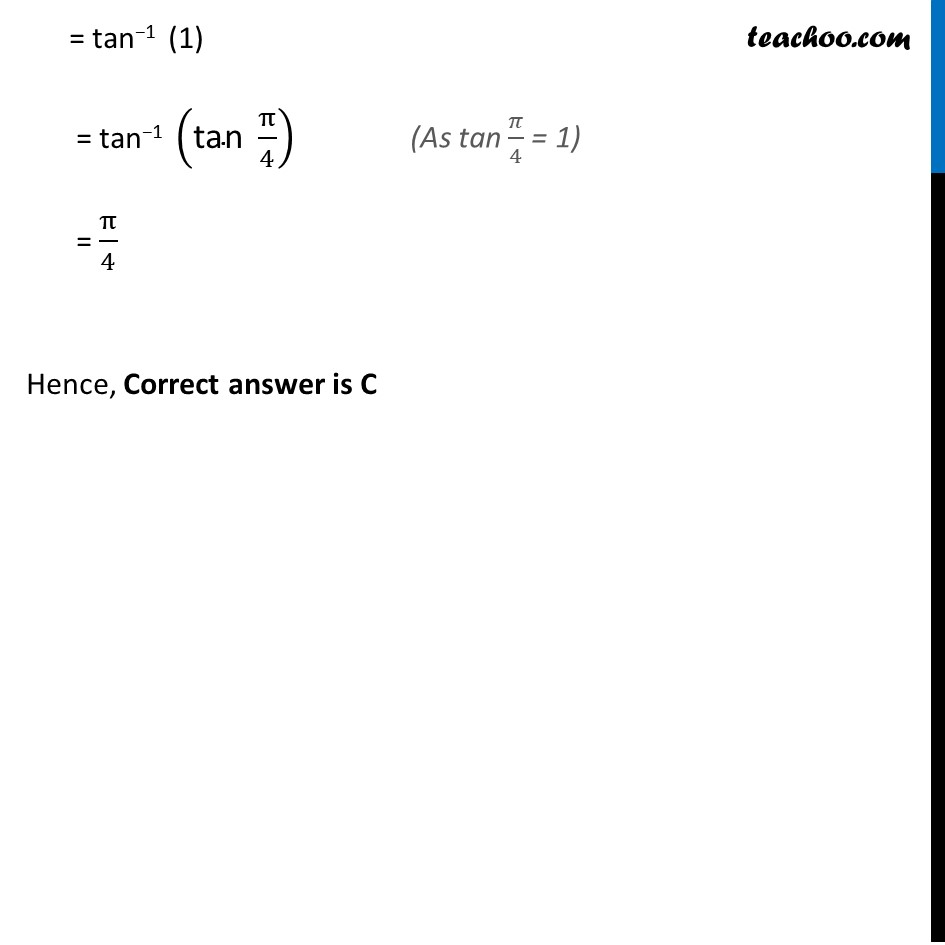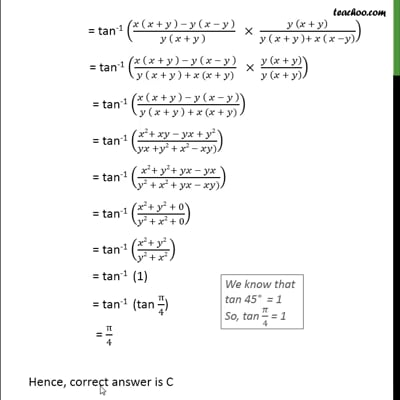Formulae based

Chapter 2 Class 12 Inverse Trigonometric Functions
Concept wiseThis video is only available for Teachoo black users

Solve all your doubts with Teachoo Black (new monthly pack available now!)

### Transcript

Misc 17 Solve tan−1 (x/y) – tan−1 (x − y)/(x + y) is equal to (A) π/2 (B) π/3 (C) π/4 (D) (−3π)/4 We know that tan–1 x – tan–1 y = tan–1 ((𝐱 − 𝐲)/(𝟏 + 𝐱𝐲)) tan−1 x/y – tan−1 ((x − y)/(x + y)) = tan−1 [(x/y − (x − y )/(x + y))/(1 + (x/y) ((x − y )/(x + y)) )] Replacing x by 𝑥/𝑦 & y by (x − y)/(x + y) = tan−1 (((𝑥 ( 𝑥 + 𝑦 ) − 𝑦 ( 𝑥 − 𝑦 ))/(𝑦 ( 𝑥 + 𝑦 ) ))/((𝑦 ( 𝑥 + 𝑦 )+ 𝑥 ( 𝑥 − 𝑦))/(𝑦 ( 𝑥 + 𝑦) ))) = tan−1 ((𝑥 ( 𝑥 + 𝑦 ) − 𝑦 ( 𝑥 − 𝑦 ))/(𝑦 ( 𝑥 + 𝑦 ) ) × (𝑦 (𝑥 + 𝑦))/(𝑦 ( 𝑥 + 𝑦 )+ 𝑥 ( 𝑥 −𝑦) )) = tan−1 ((𝑥 ( 𝑥 + 𝑦 ) − 𝑦 ( 𝑥 − 𝑦 ))/(𝑦 ( 𝑥 + 𝑦 ) + 𝑥 (𝑥 + 𝑦)) × (𝑦 (𝑥 + 𝑦))/(𝑦 (𝑥 + 𝑦) )) = tan−1 ((𝑥 ( 𝑥 + 𝑦 ) − 𝑦 ( 𝑥 − 𝑦 ))/(𝑦 ( 𝑥 + 𝑦 ) + 𝑥 (𝑥 + 𝑦))) = tan−1 ((𝑥2+ 𝑥𝑦 − 𝑦𝑥 + 𝑦2)/(𝑦𝑥 +𝑦2 + 𝑥2 − 𝑥𝑦))) = tan−1 ((𝑥2+ 𝑦2+ 𝑦𝑥 − 𝑦𝑥)/(𝑦2 + 𝑥2 + 𝑦𝑥 − 𝑥𝑦))) = tan−1 ((𝑥2+ 𝑦2 )/(𝑦2 + 𝑥2 )) = tan−1 (1) = tan−1 ("tan " π/4) = π/4 Hence, Correct answer is C (As tan 𝜋/4 = 1)